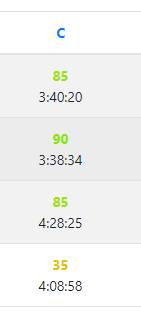## 背景## 推导

\begin{aligned} &\sum_{i=1}^{r} a_i\\=&\sum_{i=1}^r\sum_{j=1}^i b_j\\=&\sum_{i=1}^r b_i\times(r-i+1) \\=&\sum_{i=1}^r b_i\times (r+1)-\sum_{i=1}^r b_i\times i \end{aligned}